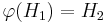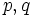# No subgroup property between normal Sylow and subnormal or between Sylow retract and retract is conditionally lattice-determined

## Statement

It is possible to have a group$G$, a lattice automorphism$\varphi: L(G) \to L(G)$ of the lattice of subgroups, and subgroups$H_1, H_2$ of$G$ with$\varphi(H_1) = H_2$, such that:

### Property-theoretic implication

No subgroup property of any of the following kinds is a conditionally lattice-determined subgroup property:

## Proof

### General example

Choose primes$p,q$ such that$q$ divides$p - 1$. Let$G$ be the semidirect product of the group of order$p$ by the subgroup of order$q$ in its automorphism group.$G$ is a group of order$pq$. Its lattice has size$p + 3$, including the trivial subgroup, whole group, and$p+1$ intermediate mutually incomparable subgroups, one of order$p$ and$p$ of order$q$.

Let$H_1$ be the subgroup of order$p$ and$H_2$ be any subgroup of order$q$. The map from$L(G)$ to itself interchanging$H_1$ and$H_2$ is a lattice automorphism, and it interchanges the two subgroups. Also,$H_1$ and$H_2$ satisfy the stated conditions, completing the proof.

### Smallest example

The smallest example is obtained by setting$p = 3, q = 2$, giving:

•$G$ equals symmetric group:S3
•$H_1$ equals A3 in S3
•$H_2$ equals S2 in S3

For more on the subgroup structure, see subgroup structure of symmetric group:S3.

The lattice of subgroups is also shown below: